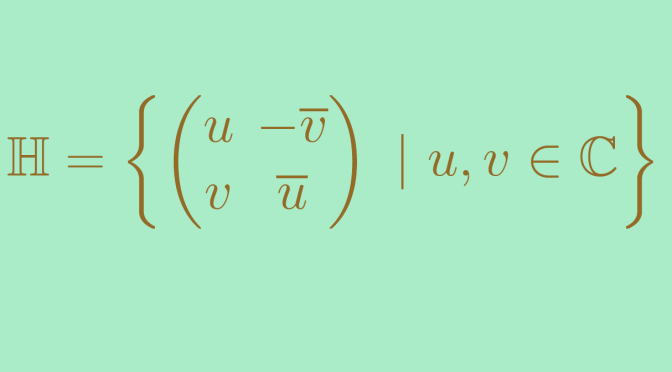# The skew field of Hamilton’s quaternions

We give here an example of a division ring which is not commutative. According to Wedderburn theorem every finite division ring is commutative. So we must turn to infinite division rings to find a non-commutative one, i.e. a skew field.

Let’s introduce the skew field of the Hamilton’s quaternions $\mathbb H = \left\{\begin{pmatrix} u & -\overline{v} \\ v & \overline{u} \end{pmatrix} \ | \ u,v \in \mathbb C\right\}$

### $$\mathbb H$$ is a subring of $$\mathcal M_2(\mathbb C)$$ (the set of matrices of dimension $$2$$ over $$\mathbb C$$)

One can easily verify that the identity matrix is an element of $$\mathbb H$$ taking $$(u,v)=(1,0)$$. Also the sum and the product of two elements of $$\mathbb H$$ belong to $$\mathbb H$$.

### The non-zero elements of $$\mathbb H$$ are invertible

Take $$(0,0) \neq (u,v) \in \mathbb C^2$$. The determinant of $$A=\begin{pmatrix} u & -\overline{v} \\ v & \overline{u} \end{pmatrix}$$ is $$\delta=\det A = \vert u \vert^2 + \vert v \vert^2 >0$$. Hence $$A$$ is invertible and $A^{-1}=\frac{1}{\delta}\begin{pmatrix} \overline{u} & \overline{v} \\ -v & u \end{pmatrix}$

Hence $$\mathbb H$$ is a division ring.

### $$\mathbb H$$ is not commutative

We denote $$\textbf{id}=\begin{pmatrix} 1 & 0 \\ 0 & 1 \end{pmatrix}$$,
$$i=\begin{pmatrix} i & 0 \\ 0 & -i \end{pmatrix}$$, $$j=\begin{pmatrix} 0 & 1 \\ -1 & 0 \end{pmatrix}$$ and $$k=\begin{pmatrix} 0 & i \\ i & 0 \end{pmatrix}$$. All are elements of $$\mathbb H$$. The identities hold $i^2=j^2=k^2=ijk=-1$ as well as following ones
$ij=-ji=k$ $jk=-kj=i$ $ki=-ik=j$
proving that $$\mathbb H$$ is not commutative. $$\mathbb H$$ is a skew field.

### $$\mathbb H$$ is a vector space of dimension $$4$$ over $$\mathbb R$$

One can verify that any matrix $$P=\begin{pmatrix} a+bi & c+di \\ -c+di & a-bi \end{pmatrix}$$ of $$\mathbb H$$ (with $$a,b,c,d \in \mathbb R^4$$) can be written $P=a \textbf{id} + bi +cj +dk$ As $$(\textbf{id}, i, j, k)$$ is an independent family, it is a basis of $$\mathbb H$$. Hence the result. One can also verify that $$\mathbb R \textbf{id}$$ is included in $$\mathbb H$$ center.